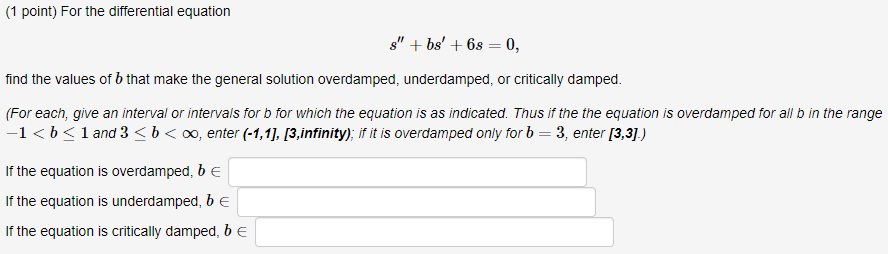# Question (1 point) For the differential equation s" + bs' +63 = 0, find the values of b that make the general solution overdamped, underdamped, or critically damped. (For each, give an interval or intervals for b for which the equation is as indicated. Thus if the the equation is overdamped for all b in the range -1<b< 1 and 3 <b<, enter (-1,1], [3,infinity); if it is overdamped only for b = 3, enter [3,3].) If the equation is overdamped, be If the equation is underdamped, be If the equation is critically damped, be